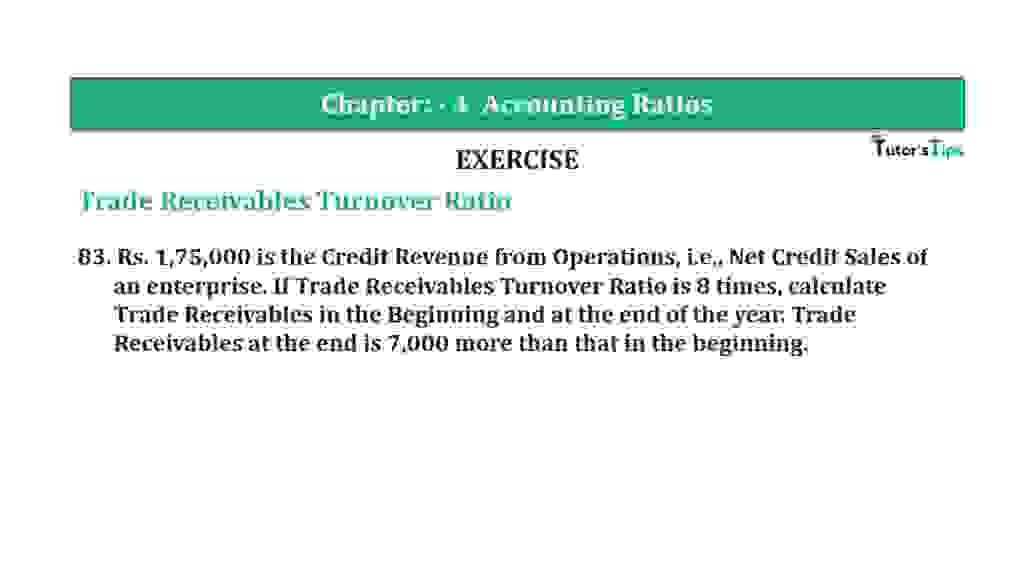# Question 83 Chapter 4 of +2-B – T.S. Grewal 12 ClassQuestion 83 Chapter 4 of +2-B

Interest Coverage Ratio

83. Rs. 1,75,000 is the Credit Revenue from Operations, i.e., Net Credit Sales of an enterprise. If Trade Receivables Turnover Ratio is 8 times, calculate Trade Receivables in the Beginning and at the end of the year. Trade Receivables at the end is 7,000 more than that in the beginning.

### The solution of Question 83 Chapter 4 of +2-B: –

 Trade Receivable Turnover Ratio = Net Credit Sales Average Trade Receivable
 8 = Rs. 1,75,000 Average Trade Receivable
 Average Trade Receivable = Rs. 1,75,000 8 = Rs. 21,875
 Opening Trade Receivables = X Closing Trade Receivables = X + Rs. 7,000

 Average Trade Receivables = Opening Trade Receivables +Closing Trade Receivables 2
 Rs. 21,875 = X + (X + Rs. 7,000) 2
 Rs. 21,875 x 2 = 2x + Rs. 7,000) Rs. 43,750 = 2x + Rs. 7,000) 2x = Rs. 43,750 – Rs. 7,000

 X = Rs. 36,750 2 = Rs. 18,375
 Opening Trade Receivables = Rs. 18,375 Closing Trade Receivables = Rs. 25,375

Balance Sheet: Meaning, Format & Examples

Thanks, Please Like and share with your friends

Comment if you have any question.

Also, Check out the solved question of previous Chapters: –

### T.S. Grewal’s Double Entry Book Keeping (Vol. II: Accounting for Companies)T.S. Grewal’s Analysis of Financial Statements